Select Page

### CAT 2022 Mensuration Practice Exercise-5

With the help of this CAT Mensuration test, you have a chance to go through the basic problems based on Mensuration. This section provides you with the ultimate test to check your level of learning for this topic. This set of test consists of problems based on some theorems, formulas and logic. Practice these to get a final overview of the whole topic.

Question 1: In triangle ABC, points P, Q and R are the mid-points of sides AB, BC and CA respectively. If area of triangle ABC, is 20 sq. units, find the area of triangle PQR.

(a) 10 sq. units
(b) 53  sq. units
(c) 5 sq. units
(d) None of these

Answer: (c)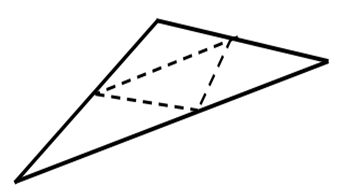Midpoint theorem states that the line joining the midpoints of two

sides of a triangle is parallel to and half of the third side.

Let the sides of the bigger triangle be a, b and c. then the corresponding sides of the smaller triangle will be c/2, b/2 and a/2.

We know that area of a triangle can be represented by its sides by Heron’s formula  –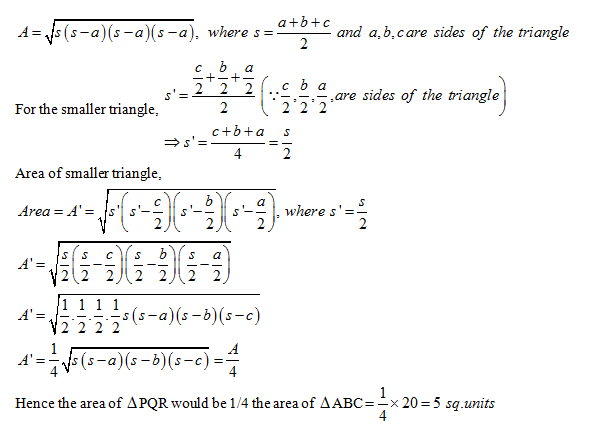Question 2: The adjoining figures show a set of concentric squares. If the diagonal of the innermost square is 2 units, and if the distance between the corresponding comers of any two successive squares is 1unit, find the difference between the areas of the eight and the seventh squares, counting from the innermost square.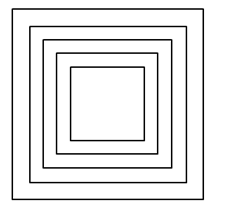(a) 102sq. unit
(b) 30 sq. units
(c) 352sq. units
(d) None of these

Given that the diagonal of the innermost square is 2 units and the distance between the corners of adjacent is 1 unit. Therefore as is clear the diagonal of the next square will be 2 units more than the innermost square. So we can say that,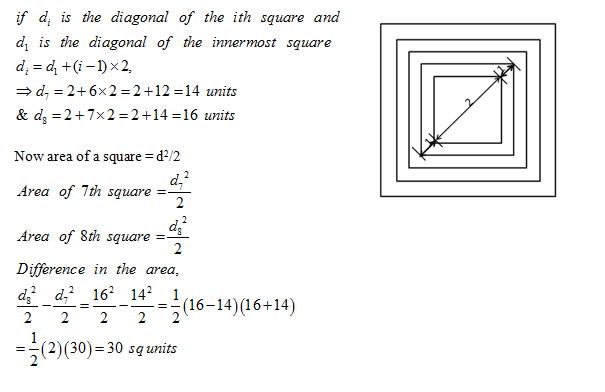Question 3: AB is the diameter of the given circle, while points C and D lie on the circumference as shown. If AB is 15 cm, AC is 12 cm and BD is 9 cm, find the area of quadrilateral ACBD.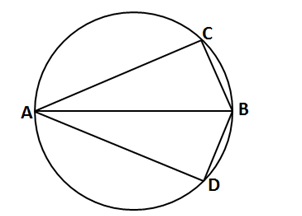(a) 54 sq. cm
(b) 216 sq. cm
(c) 162 sq. cm
(d) None of these

According to theorem, the diameter of the circle creates an angle of 90˚ at each point on the circumference of the circle. Hence angle ACB= angle ADB = 90o

In ABC, AB = 15, AC = 12 and angle ACB = 90˚, Applying Pythagoras theorem in triangle ABC

AC2 + BC2 = AB2 =>BC2 = AB2 – AC2 = 225 – 144 = 81 => BC = 9

In triangle ADB, AB = 15, BD = 9 and angle ADB = 90, Therefore AD = AC = 12

=>Area of triangle ABC = Area of triangle BAD = ½ × AC × BC = ½ × AD × BD = ½ × 12 × 9 = 54 cm2

Area of quadrilateral ACBD = Area of triangle ABC + Area of triangle BAD = 54 + 54 = 108 cm2

Question 4: The sum of the areas of two circles, which touch each other externally, is 153π ft. If the sum of their radii is 15, find the ratio of the larger to the smaller radius.

(a) 4
(b) 2
(c) 3
(d) None of these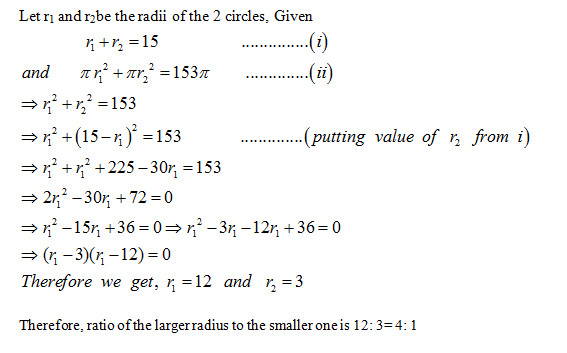Question 5: Four identical coins are placed in a square. For each coin, the ratio of area to circumference is same as the ratio of circumference to area. Then, find the area of the square that is not covered by the coins.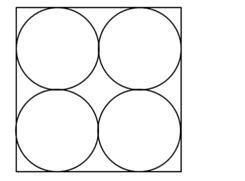(a) 16 ( π–1)
(b) 16 (16–π )
(c) 16 (4–π )
(d) 16(4-π/2)

Let R be the radius of each circle,πR2 is the area and 2πR is circumference. Then by given condition,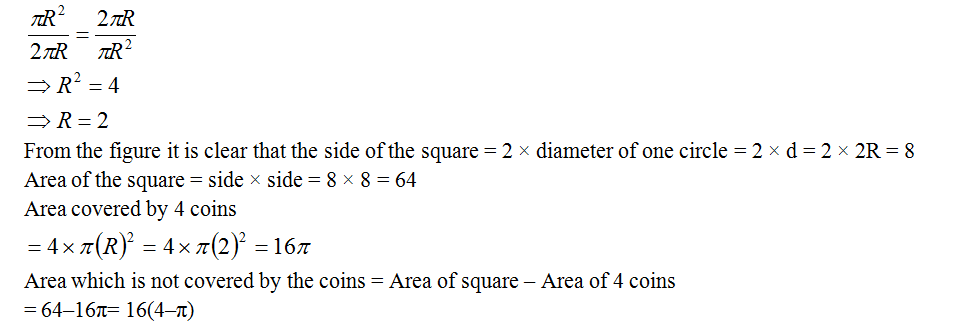Extra tips for CAT Mensuration Test:

• The purpose of this CAT Mensuration test was to highlight the important concepts you need to go through. Revise the basic such as heron’s formula, Pythagoras theorem, Midpoint theorem, etc.
• Analyze the questions and draw the diagrams accordingly. Draw the shapes in proportion to each other to avoid any confusion related to how the various shapes are interconnected.
• In formulas where П is involved we can find the answer by checking the given options for divisibility of 11. This can only be applied to questions where 1 shape is specified.
• Solve tons of questions to grab the techniques and formulas. At the end of the day, practice is best solution for improving in Mathematics.

Get Posts Like This Sent to your Email
Updates for Free Live sessions and offers are sent on mail. Don't worry: we do not send too many emails..:)
Get Posts Like This Sent to your Email
Updates for Free Live sessions and offers are sent on mail. Don't worry: we do not send too many emails..:)